# ggeasy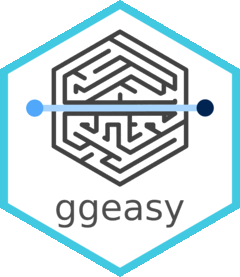You know how to make `ggplot2` graphics, right? No worries. Piece of cake.

Now, can you please rotate the `x` axis labels to vertical?`ggeasy` is here to make that a little easier.

## Installation

You can install the latest released version of `ggeasy` from CRAN with:

``install.packages("ggeasy")``

or the bleeding-edge development version from GitHub with

``````# install.packages("remotes")
remotes::install_github("jonocarroll/ggeasy")``````

## Reference

See the `pkgdown` site.

[@amrrs](https://github.com/amrrs) a.k.a. [@1littlecoder](https://twitter.com/1littlecoder) has produced a video walkthrough using `ggeasy` which covers some of the major features:## Examples

``````library(ggplot2)
library(ggeasy)

# rotate x axis labels
ggplot(mtcars, aes(hp, mpg)) +
geom_point() +
easy_rotate_x_labels()``````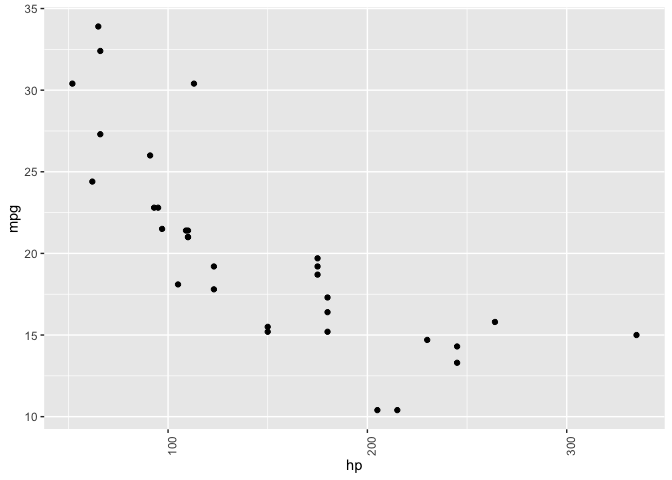``````# rotate y axis labels
ggplot(mtcars, aes(hp, mpg)) +
geom_point() +
easy_rotate_y_labels()``````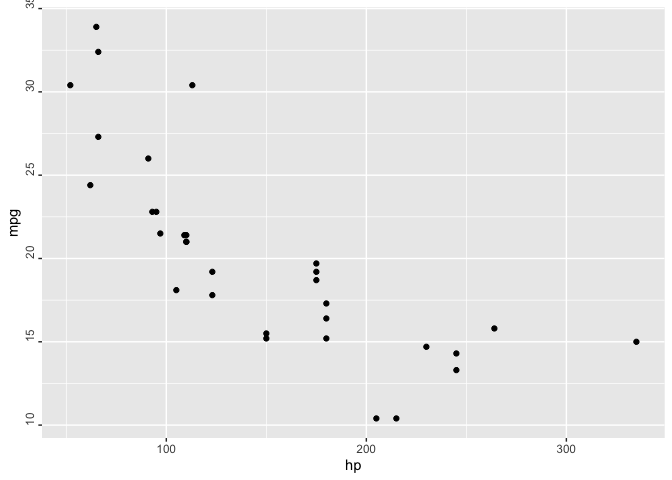``````# remove 'size' legend
ggplot(mtcars, aes(wt, mpg, colour = cyl, size = hp)) +
geom_point() +
easy_remove_legend(size)``````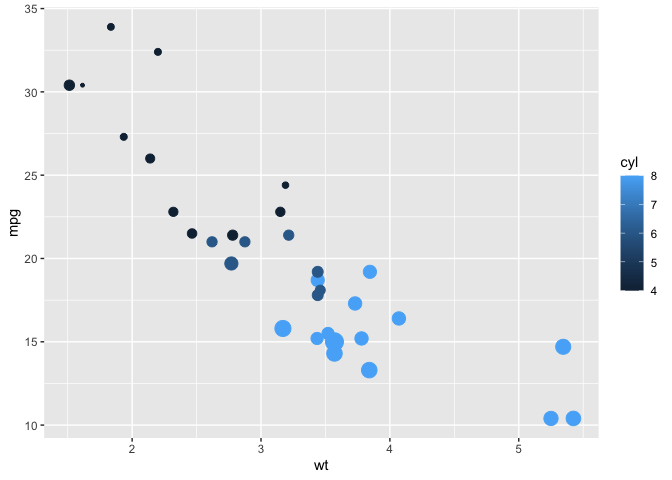``````# make the x axis labels larger
ggplot(mtcars, aes(mpg, hp)) +
geom_point() +
easy_x_axis_labels_size(22)``````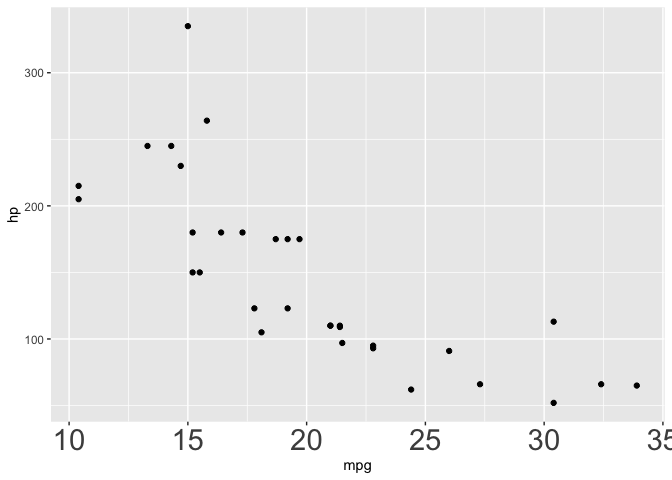``````# make all the text red
ggplot(mtcars, aes(mpg, hp)) +
geom_point(aes(fill = gear)) +
easy_all_text_color("red")``````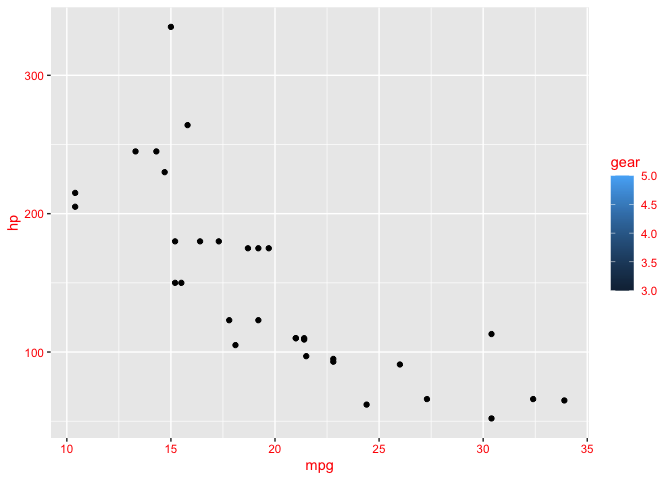``````# remove just x axis
ggplot(mtcars, aes(wt, mpg)) +
geom_point() +
easy_remove_x_axis()``````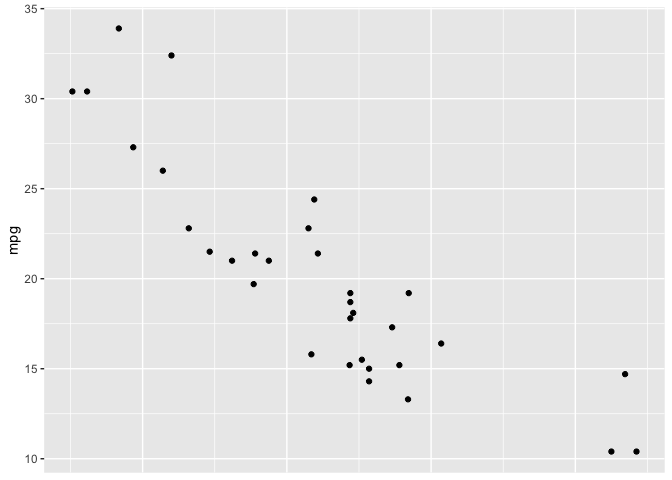``````# remove y axis ticks
ggplot(mtcars, aes(wt, mpg)) +
geom_point() +
easy_remove_y_axis(what = "ticks")``````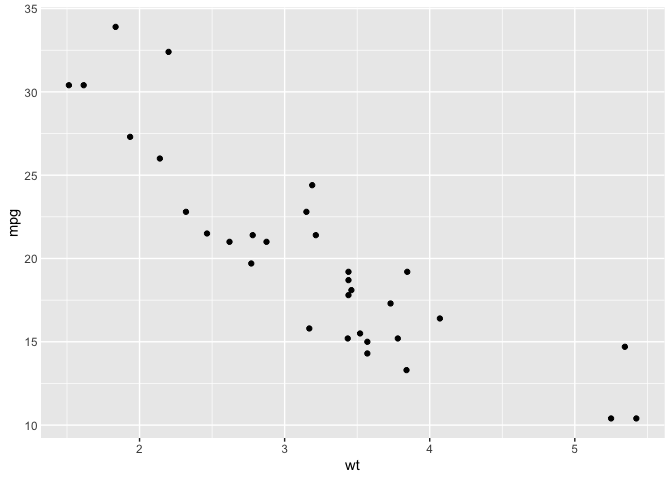``````# move legends to bottom
ggplot(mtcars, aes(wt, mpg, colour = cyl, size = hp)) +
geom_point() +
easy_move_legend("bottom")``````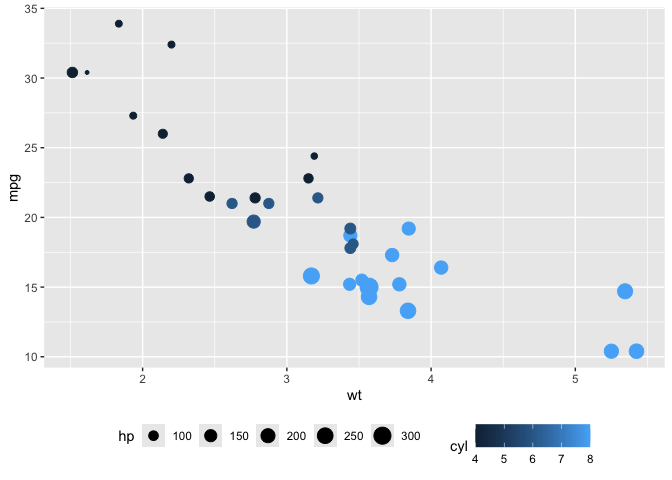``````# move legend to left side
ggplot(mtcars, aes(wt, mpg, colour = cyl, size = hp)) +
geom_point() +
easy_legend_at("left")``````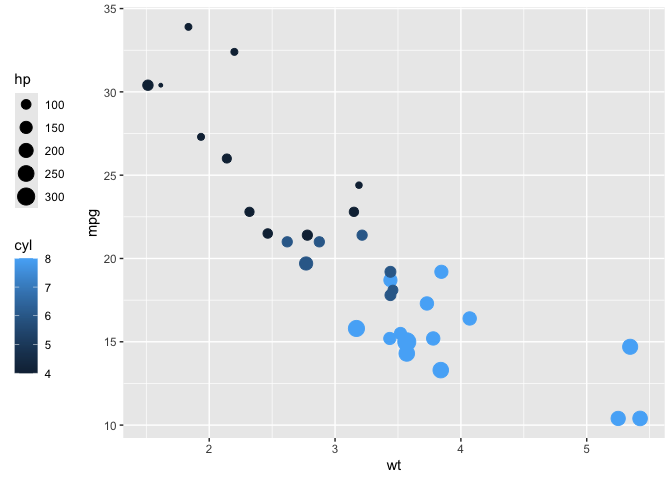``````# Make legends horizontal
ggplot(mtcars, aes(wt, mpg, colour = cyl, size = hp)) +
geom_point() + easy_rotate_legend("horizontal")``````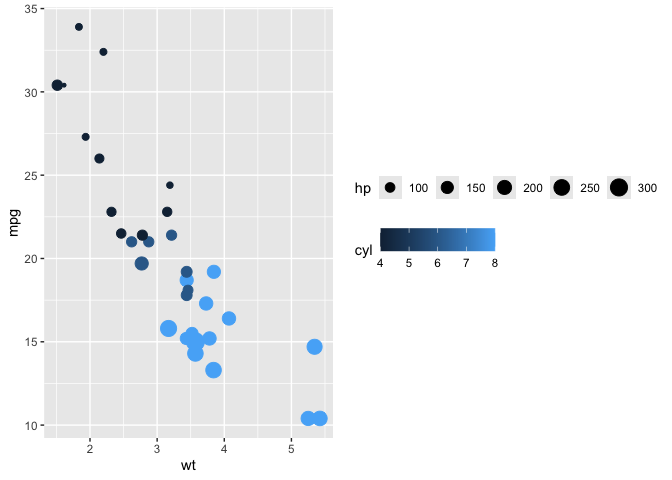``````# use labelled variables
iris_labs <- iris
labelled::var_label(iris_labs\$Species) <- "Flower\nSpecies"
labelled::var_label(iris_labs\$Sepal.Length) <- "Length of Sepal"
iris_labs_2 <- iris_labs
labelled::var_label(iris_labs_2\$Species) <- "Sub-genera"

# use variable labels automatically
ggplot(iris_labs, aes(x = Sepal.Length, y = Sepal.Width)) +
geom_line(aes(colour = Species)) +
geom_point(data = iris_labs_2, aes(fill = Species), shape = 24) +
easy_labs()``````These functions will try to teach you the ‘official’ way to achieve these goal, usually via the `teach` argument (where implemented)

``````ggplot(mtcars, aes(hp, mpg)) +
geom_point() +
easy_rotate_y_labels(angle = "startatbottom", teach = TRUE)
#> easy_rotate_y_labels call can be substituted with:
#> theme(axis.text.y = element_text(angle = 90, hjust = 0))````````````ggplot(mtcars, aes(wt, mpg)) +
geom_point() +
easy_remove_y_axis(what = "ticks", teach = TRUE)
#> easy_remove_y_axis call can be substituted with:
#> theme(axis.ticks.y = element_blank())``````## Credits

Many thanks to [Sébastien Rochette (@statnmap)](https://statnmap.com/) for the design and execution of the hex logo.